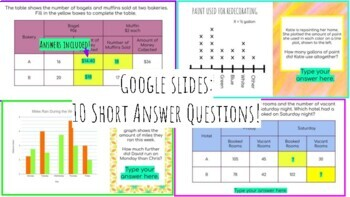# Tables, Line Plots & Bar Graphs - Google Slides & Printable PDF! Dozens of ?sSubject
Resource Type
Format
Zip (719 KB|20+)
Standards
\$3.00
\$3.00

### Description

Common Core Aligned! Many of these questions model past NYS Regents exams for grades 4 & 5. (A little tricky for 4th, I used as enrichment only)

THE PRODUCT:

1. Google Slides - 20 questions! 10 multiple choice, 10 short answer.

2. Printable PDF with 4 pages of questions. +30 questions. (I used the PDF as four separate worksheets for practice & hw)

3. Answer keys included for both!

MATH GOALS:

Focus:

- Interpreting line plots

- Interpreting & completing tables

- Interpreting bar graphs

Skills Required:
- Adding, subtracting & multiplying decimals, fractions & coins

- Mean, median, mode & range

- Percentages (from fractions - will convert evenly)

- Converting hours to minutes

BONUS - Some questions on the PDF encourage the (optional) use of bar modeling! Answer keys provide example bar models.

THE GOOGLE SLIDES PORTION OF THIS PRODUCT WOULD WORK AS A WONDERFUL FULL-YEAR REVIEW! HITS MANY OF THE MAJOR 5TH GRADE STANDARDS. I used with all 5th graders & 4th graders in the enrichment group.

Total Pages
20+
Included
Teaching Duration
1 hour
Report this Resource to TpT
Reported resources will be reviewed by our team. Report this resource to let us know if this resource violates TpT’s content guidelines.

### Standards

to see state-specific standards (only available in the US).
Solve word problems involving addition and subtraction of fractions referring to the same whole, including cases of unlike denominators, e.g., by using visual fraction models or equations to represent the problem. Use benchmark fractions and number sense of fractions to estimate mentally and assess the reasonableness of answers. For example, recognize an incorrect result 2/5 + 1/2 = 3/7, by observing that 3/7 < 1/2.
Add and subtract fractions with unlike denominators (including mixed numbers) by replacing given fractions with equivalent fractions in such a way as to produce an equivalent sum or difference of fractions with like denominators. For example, 2/3 + 5/4 = 8/12 + 15/12 = 23/12. (In general, 𝘢/𝘣 + 𝘤/𝘥 = (𝘢𝘥 + 𝘣𝘤)/𝘣𝘥.)
Add and subtract mixed numbers with like denominators, e.g., by replacing each mixed number with an equivalent fraction, and/or by using properties of operations and the relationship between addition and subtraction.
Understand addition and subtraction of fractions as joining and separating parts referring to the same whole.
Multiply a whole number of up to four digits by a one-digit whole number, and multiply two two-digit numbers, using strategies based on place value and the properties of operations. Illustrate and explain the calculation by using equations, rectangular arrays, and/or area models.